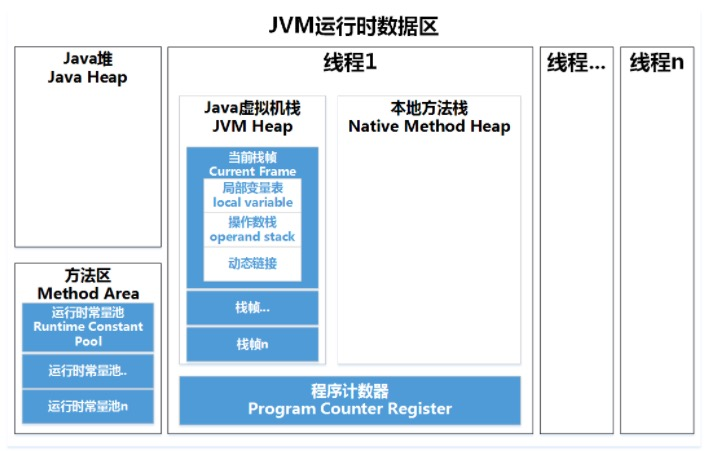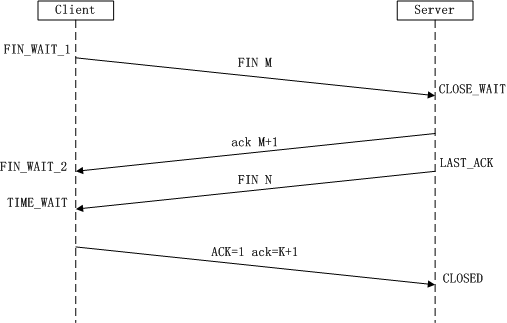# 一、Java

## 说明类中public和private的基本区别

public Y Y Y Y
protected Y Y Y N
default Y Y N N
private Y N N N

## volatile底层原理

volatile是变量修饰符，其修饰的变量具有可见性，Java的做法是将该变量的操作放在寄存器或者CPU缓存上进行，之后才会同步到主存，使用volatile修饰符的变量是直接读写主存，volatile不保证原子性，同时volatile禁止指令重排。

# 二、JVM

## JVM内存分布Java虚拟机栈:每个 Java 方法在执行的同时会创建一个栈帧用于存储局部变量表、操作数栈、常量池引用等信息。每一个方法从调用直至执行完成的过程，就对应着一个栈帧在 Java 虚拟机栈中入栈和出栈的过程。

Java堆:几乎所有对象实例都在这里分配内存。是垃圾收集的主要区域（"GC 堆"），虚拟机把 Java 堆分成以下三块：

• 新生代
• 老年代
• 永久代

# 三、数据结构与算法

## 两个栈实现一个队列

import java.util.Stack;

/**
* 用两个栈实现队列
* 用两个栈来实现一个队列，完成队列的Push和Pop操作。 队列中的元素为int类型。
* 思路：
* 栈A用来作入队列，栈B用来出队列
* 当栈B为空时，栈A全部出栈到栈B,栈B再出栈（即出队列）
*/
public class Solution18 {
public static void main(String[] args) {
Solution18 solution18 = new Solution18();
solution18.push(1);
solution18.push(2);
System.out.println(solution18.pop());
solution18.pop();
}

Stack<Integer> stack1 = new Stack<Integer>();
Stack<Integer> stack2 = new Stack<Integer>();

public void push(int node) {
stack1.push(node);//stack1负责入队
}

public int pop() {
if (stack1.empty() && stack2.empty()) {
throw new RuntimeException("队列为空");
}
if (stack2.empty()) {
while (!stack1.empty()) {
stack2.push(stack1.pop());
}
}
return stack2.pop();//stcak2负责出队
}
}


## 堆排序

public class HeapSort {
/**
* 构建大顶堆
*/
public static void adjustHeap(int[] a, int i, int len) {
int temp, j;
temp = a[i];
for (j = 2 * i; j < len; j *= 2) {// 沿关键字较大的孩子结点向下筛选
if (j < len && a[j] < a[j + 1])
++j; // j为关键字中较大记录的下标
if (temp >= a[j])
break;
a[i] = a[j];
i = j;
}
a[i] = temp;
}

public static void heapSort(int[] a) {
int i;
for (i = a.length / 2 - 1; i >= 0; i--) {// 构建一个大顶堆
}
for (i = a.length - 1; i >= 0; i--) {// 将堆顶记录和当前未经排序子序列的最后一个记录交换
int temp = a;
a = a[i];
a[i] = temp;
adjustHeap(a, 0, i - 1);// 将a中前i-1个记录重新调整为大顶堆
}
}

public static void main(String[] args) {
int a[] = { 51, 46, 20, 18, 65, 97, 82, 30, 77, 50 };
heapSort(a);
System.out.println(Arrays.toString(a));
}
} 

## 写一个 “123” 转为123

public class Solution {
public static void main(String[] args) {
Solution solution = new Solution();
String s1 = "+2147483647";
String s2 = "1a33";
System.out.println(solution.StrToInt(s2));
}

/**
* 判断字符串为空，长度为0,判断字符串转换后上下界是否溢出
*
* @param str
* @return
*/
public int StrToInt(String str) {
//判断字符串是否为空，长度是否为0
if (str == null || str.length() == 0) {
return 0;
}
int start;
int tag;//1表示+ 0表示-
if (str.charAt(0) == '+') {
start = 1;
tag = 1;
} else if (str.charAt(0) == '-') {
start = 1;
tag = 0;
} else {
start = 0;
tag = 1;
}
long result = 0;
for (int i = start; i < str.length(); i++) {
char temp = str.charAt(i);
if (temp >= '0' && temp <= '9') {
result = result * 10 + (temp - '0');
if (tag == 1 && result > Integer.MAX_VALUE) throw new RuntimeException("上溢出");
if (tag == 0 && result < Integer.MIN_VALUE) throw new RuntimeException("下溢出");
} else {
return 0;
}
}

if (tag == 0) {
return (int) (-1 * result);
} else {
return (int) result;
}
}
}


## 水仙花数

public class Solution {

public static void main(String[] args) {
for (int i = 100; i <1000 ; i++) {
int firstNum = i/100;
int secondNum = i/10%10;
int thirdNum = i%10;
if(firstNum*firstNum*firstNum + secondNum*secondNum*secondNum+thirdNum*thirdNum*thirdNum == i){
System.out.println("水仙花数为：" + i);
}
}
}
}


# 四、网络

## TCP四次挥手1. 第一次挥手：Client发送一个FIN，用来关闭Client到Server的数据传送，Client进入FIN_WAIT_1状态。
2. 第二次挥手：Server收到FIN后，发送一个ACK给Client，确认序号为收到序号+1（与SYN相同，一个FIN占用一个序号），Server进入CLOSE_WAIT状态。
3. 第三次挥手：Server发送一个FIN，用来关闭Server到Client的数据传送，Server进入LAST_ACK状态。
4. 第四次挥手：Client收到FIN后，Client进入TIME_WAIT状态，接着发送一个ACK给Server，确认序号为收到序号+1，Server进入CLOSED状态，完成四次挥手。

## GET和POST的区别

GET 请求：

• GET 请求可被缓存
• GET 请求保留在浏览器历史记录中
• GET 请求可被收藏为书签
• GET 请求不应在处理敏感数据时使用
• GET 请求有长度限制
• GET 请求只应当用于取回数据

POST 请求 ：

• POST 请求不会被缓存
• POST 请求不会保留在浏览器历史记录中
• POST 不能被收藏为书签
• POST 请求对数据长度没有要求

## 写出几个IP保留地址

0.0.0.0 不清楚主机和目的网络集合

255.255.255.255 限制广播地址

127.0.0.1 本机地址，用于测试

224.0.0.0到239.255.255.255 组播地址

# 六、设计模式

## 手写一个单例模式。

public class Singleton {
private volatile static Singleton instance = null;

private Singleton() {

}

/**
* 当第一次调用getInstance()方法时，instance为空，同步操作，保证多线程实例唯一
* 当第一次后调用getInstance()方法时，instance不为空，不进入同步代码块，减少了不必要的同步
*/
public static Singleton getInstance() {
if (instance == null) {
synchronized (Singleton.class) {
if (instance == null) {
instance = new Singleton();
}
}
}
return instance;
}
}

# 八、框架

## springmvc流程

posted @ 2018-10-18 19:50  武培轩  阅读(...)  评论(...编辑  收藏